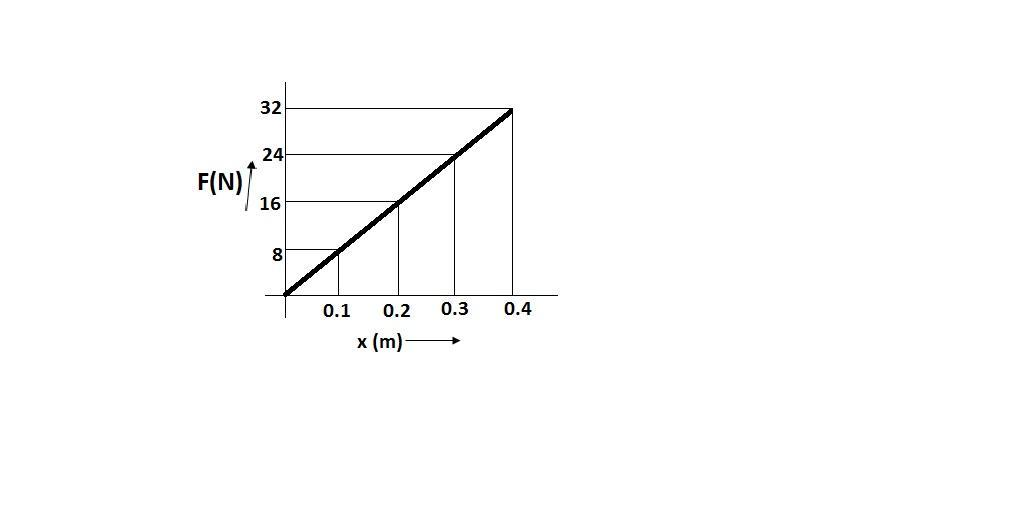Courses
Courses for Kids
Free study material
Offline Centres
MoreLast updated date: 03rd Dec 2023
Total views: 345.3k
Views today: 9.45k

# The graph $F - x$ is given, find the compression produced in the spring when a body of mass $5kg$ moving with velocity $8m/s$ hits the spring. Also calculate the force constant of the spring.Verified
345.3k+ views
Hint: To find the force constant of the spring see the graph and find the slope. Then, calculate it by putting values. Now, to calculate the compression we have to use the conservation of energy in which total energy in an isolated system remains constant. So, kinetic and potential energy become equal to each other.

From the graph in the question, we can conclude that the slope of the graph is the force constant of the spring.
$\because F = kx \\ \therefore k = \dfrac{x}{F} \\$
where, $F$ is the force
$k$ is the force constant
$x$ is the compression of the spring
The slope of a graph can be calculated when we determine the difference between coordinates of the y – axis and x – axis respectively. After this, the differences of y – coordinates and x – coordinates are divided.
If we take $\left( {0.3m,24N} \right)$ and $\left( {0.2m,16N} \right)$ coordinates from x – axis and y – axis respectively, we get –
$\implies Slope = \dfrac{{24 - 16}}{{0.3 - 0.2}} \\ \Rightarrow Slope = \dfrac{8}{{0.1}} \\ Slope = 80N/m \\$
We know that, $slope = k$
Therefore, the force constant of the spring is $80N/m$.
From the question, we can conclude that the block will continue to compress till the block comes to the rest.
Now, using the conservation of energy the potential energy of the spring and kinetic energy of the spring becomes equal to each other because according to conservation of energy in an isolated system, the total energy remains constant and is said to be conserved over time.
$\therefore U = K \cdots \left( 1 \right)$
where, $U$ is the potential energy and $K$ is the kinetic energy
We know that, for a spring
$\implies U = \dfrac{1}{2}k{x^2} \\ \implies K = \dfrac{1}{2}m{v^2} \\$
From equation $\left( 1 \right)$, we get –
$\dfrac{1}{2}k{x^2} = \dfrac{1}{2}m{v^2}$
Cancelling $\dfrac{1}{2}$ on both sides, we get –
$k{x^2} = m{v^2} \\ \implies x = v\sqrt {\dfrac{m}{k}} \cdots \left( 2 \right) \\$
According to the question, it is given that –
Velocity, $v = 8m/s$
Mass, $m = 5kg$
Putting these values in equation $\left( 2 \right)$, we get –
$\implies x = 8\sqrt {\dfrac{5}{{80}}} \\ \implies x = 8\sqrt {\dfrac{1}{{16}}} \\ \implies x = \dfrac{8}{4} = 2m \\$
Hence, compression produced by the spring after the hitting of the body is $2m$.

Note: A spring stores potential energy due to extension. Since an unextended spring does not store potential energy, it is used as the point of zero energy.  For a spring, potential energy is defined as, $U = \dfrac{1}{2}k{x^2}$ where, $x$ is the compression of the spring.